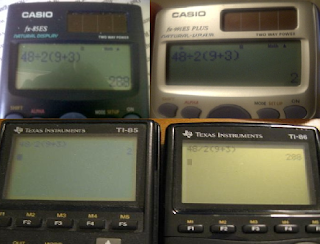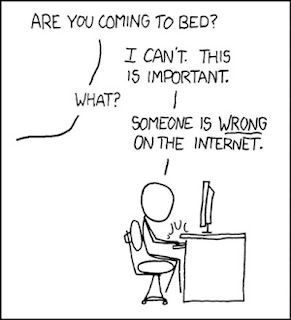### 48÷2(9+3) =

Category : advertising, graphic design, problem solving · No Comments · by Apr 12th, 2011

The Argument
This has become a viral debate online. It has spanned across facebook, blogs, and various message boards and in nearly every case people are split just about 50/50 between the answers “2” and “288”. Math teachers are split, math students are split, even the physics forum is split! People are debating this fervently. Even calculators are split on the debate:The reason for such a divided outcome is that it is a poorly devised equation. One side, the side that answers “2” (we’ll call them side A), reads it as:

Formula A:
48
__
2(9+3)

The other side, the side that answers “288” (we’ll call them side B), reads it as:

Formula B
48
__ (9+3)
2

What’s even more amusing is that both sides are using PEMDAS to back up their argument. The acronym has M before D, but this is merely a mnemonic device to remember the order of operations. Multiplication and division actually have no preference over each other as explained here:

A common technique for remembering the order of operations is the abbreviation “PEMDAS”, which is turned into the phrase “Please Excuse My Dear Aunt Sally”. It stands for “Parentheses, Exponents, Multiplication and Division, and Addition and Subtraction”. This tells you the ranks of the operations: Parentheses outrank exponents, which outrank multiplication and division (but multiplication and division are at the same rank), and these two outrank addition and subtraction (which are together on the bottom rank).

Thus, side B claims that since there is no preference between M&D and it goes left to right, then one must therefore divide first. But, then side A claims that since there is distributive property, this must be used first. This is stated here:

= 16 ÷ 2 + 1 (**)
= 16 ÷ 4 + 1
= 4 + 1
= 5

The confusing part in the above calculation is how “16 divided by 2 + 1” (in the line marked with the double-star) becomes “16 divided by 4 + 1”, instead of “8 times by 2 + 1”. That’s because, even though multiplication and division are at the same level (so the left-to-right rule should apply), parentheses outrank division, so the first 2 goes with the , rather than with the “16 divided by”. That is, multiplication that is indicated by placement against parentheses (or brackets, etc) is “stronger” than “regular” multiplication.Typesetting the entire problem in a graphing calculator verifies this hierarchy:

Using Proofs
Essentially both sides have good arguments because they are reading the problems differently. Each side has offered proofs that show that their answer is right and the other is wrong. For example, here is a proof proposed by side A that claims to prove the answer is “2”:

48÷x(9+3) = 2
48 / (x*9+x*3) = 2
48 / 12x = 2
4 / x = 2
4=2x
2=x (it works)

48÷x(9+3) = 288
48 / (x*9+x*3) = 288
48 / 12x = 288
4 / x = 288
4=288x
0.0138888888889=X (it doesn’t work)

At first glance, it seems as though the debate is over. But remember, each side is viewing the problem differently. This proof works the problem as if the problem is

48
__
2 (9+3)

But remember, side B does not view the problem this way. If you work this proof in the formula as they see it, one gets different results:

48
__ (9+3)=288
x

48(9+3)=288x
576=288x
2=x (it works)

vs

48
__ (9+3)=2
x

48(9+3)=2x
576=2x
288=x (it doesn’t work)

Using Examples:
As one can see, even in proofs, the answer still relies on how one views the problem. People have also attempted to use real life examples to show how one formula works while the other doesn’t. For example, side B has proposed this:

We know that if you take add 1 cup with 1 cup you have two cups. (1+1) = 2

If you halve two cups you get 1 cup
1÷2(2) = 1

You express this same problem mathematically with the following equation:

1÷2(1+1) = 1

From here, we apply this to both formats. First let’s use the formula that side A has used:

1
_
2(1+1) =1/4

This doesn’t equal one, which we all know is how many cups we have.

Now, let’s write it the way one that says “288” would:

1
__ (1+1)
2

1
__ (2)= 1.
2

It appears that the logical format would be:

48
__ (9+3)
2

This proves that the side B formula works and the side A formula doesn’t so the answer is “288”, right? Well not so fast. This has the same problem that the previous proofs had. The word problem used (cups) is a scenario where equation B would be needed. But remember, side A reads the equation as A. There are word problems that would fit formula A as well. It is possible to derive a life scenario where equation A would work, but equation B would not. This is yet another case of people solving different problems, which is the reason both sides can create scenarios that prove theirs to be correct and the others to be wrong. Once again, it comes down to what one perceives to be the problem.

Problem Solving

The first step in problem solving for design or advertising is to state the problem. If one accurately identifies the problem, then said person can accurately create a strong solution. The problem with both sides of this debate is that people were not convinced on what the problem was. People recognized two different problems. This example shows how one can create the wrong solution when said person does not correctly identify the problem. Likewise, in advertising, it is essential that one identifies the problem correctly in order to find the most effective solution.

Creative Opportunity?
It’s an interesting debate and I can see both sides of the argument. What I think is more interesting, however, is how passionate people have been over such an irrelevant argument. This debate is all over the internet and debates are getting heated. The internet has often become a place for debate. People debate religion, politics, sports, movies, etc. all the time. But with those issues, people are usually emotionally invested in the subject. With this subject, people don’t really have anything emotionally invested in the subject, yet they still have heated debates. People might have something to gain from convincing another to change positions on a political issue, but no one really has anything to gain from winning an argument over a math equation. But, people still engaged in this debate.People will put a lot more time and effort into an argument online than they would with people at home or in the office. The internet has provided an outlet for discussion and debate that didn’t previously exist, or not to this extent. The exciting thing about the advertising industry is that the internet (especially social media) is still relatively new and there are many ideas that have not yet been implemented or attempted. We have seen how engaged people can become in a debate and how debates over something as simple as a math problem can go viral. Wouldn’t it be interesting to start a viral debate over a brand? I’m not talking about debate in a negative way, but perhaps a debate between two good qualities of a product (an example that comes to mind is Miller Lite’s “more taste vs less filling” ads, but I’m sure a better idea could be used).

This math equation may have been posed accidentally, but let’s assume a person actually created it with the intention of trolling people. If that’s the case, then said person has not only created an equation that has been posted on many sites (sites the original poster has never even visited) and received thousands of responses on each, but has also created awareness of the brand (the math equation). If a person asked me a math equation, I would normally forget the equation after a few minutes, but since this is everywhere and I’ve read so many arguments over it, I can easily remember “48/2(9+3)”. The equation appears in google searches, on blogs, on message boards, and it is being debated to no end. Imagine if this were the case with a brand! It seems like a campaign focused on starting an online debate over a non-offensive question or statement would be an interesting way to get people to discuss the brand (either directly or indirectly). There are many ways for information to go viral online, but I haven’t seen anyone attempt to advertise a product through viral debate, which is perhaps one of the easiest way to engage people who can’t resist proving the others wrong (as shown in the comic above).